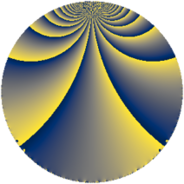Properties

 Label 230.3.hLevel $230$ Weight $3$ Character orbit 230.h Rep. character $\chi_{230}(11,\cdot)$ Character field $\Q(\zeta_{22})$ Dimension $160$ Newform subspaces $1$ Sturm bound $108$ Trace bound $0$

Related objects

Defining parameters

 Level: $$N$$ $$=$$ $$230 = 2 \cdot 5 \cdot 23$$ Weight: $$k$$ $$=$$ $$3$$ Character orbit: $$[\chi]$$ $$=$$ 230.h (of order $$22$$ and degree $$10$$) Character conductor: $$\operatorname{cond}(\chi)$$ $$=$$ $$23$$ Character field: $$\Q(\zeta_{22})$$ Newform subspaces: $$1$$ Sturm bound: $$108$$ Trace bound: $$0$$

Dimensions

The following table gives the dimensions of various subspaces of $$M_{3}(230, [\chi])$$.

Total New Old
Modular forms 760 160 600
Cusp forms 680 160 520
Eisenstein series 80 0 80

Trace form

 $$160 q - 32 q^{4} + 8 q^{6} - 64 q^{9} + O(q^{10})$$ $$160 q - 32 q^{4} + 8 q^{6} - 64 q^{9} - 24 q^{13} - 64 q^{16} + 220 q^{17} + 32 q^{18} + 132 q^{19} + 132 q^{21} - 4 q^{23} + 16 q^{24} + 80 q^{25} - 272 q^{26} - 564 q^{27} - 200 q^{29} - 280 q^{31} + 160 q^{35} - 128 q^{36} + 16 q^{39} + 332 q^{41} + 176 q^{43} + 124 q^{46} + 128 q^{47} - 236 q^{49} - 528 q^{51} - 48 q^{52} - 352 q^{53} + 84 q^{54} - 440 q^{55} + 264 q^{56} + 1452 q^{57} + 720 q^{58} + 280 q^{59} + 616 q^{61} + 728 q^{62} + 1100 q^{63} - 128 q^{64} + 176 q^{66} + 88 q^{67} + 268 q^{69} + 120 q^{70} - 236 q^{71} - 288 q^{72} - 328 q^{73} - 528 q^{74} - 1044 q^{77} - 888 q^{78} - 440 q^{79} - 1008 q^{81} - 1168 q^{82} - 1628 q^{83} - 352 q^{84} - 60 q^{85} - 396 q^{86} + 680 q^{87} + 88 q^{89} - 8 q^{92} - 856 q^{93} + 216 q^{94} + 160 q^{95} + 32 q^{96} - 264 q^{97} - 256 q^{98} - 1056 q^{99} + O(q^{100})$$

Decomposition of $$S_{3}^{\mathrm{new}}(230, [\chi])$$ into newform subspaces

Label Dim. $$A$$ Field CM Traces $q$-expansion
$a_{2}$ $a_{3}$ $a_{5}$ $a_{7}$
230.3.h.a $160$ $6.267$ None $$0$$ $$0$$ $$0$$ $$0$$

Decomposition of $$S_{3}^{\mathrm{old}}(230, [\chi])$$ into lower level spaces

$$S_{3}^{\mathrm{old}}(230, [\chi]) \cong$$ $$S_{3}^{\mathrm{new}}(23, [\chi])$$$$^{\oplus 4}$$$$\oplus$$$$S_{3}^{\mathrm{new}}(46, [\chi])$$$$^{\oplus 2}$$$$\oplus$$$$S_{3}^{\mathrm{new}}(115, [\chi])$$$$^{\oplus 2}$$